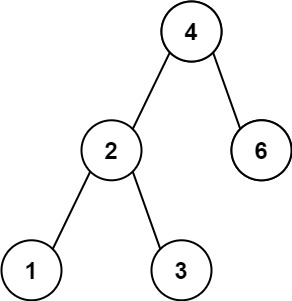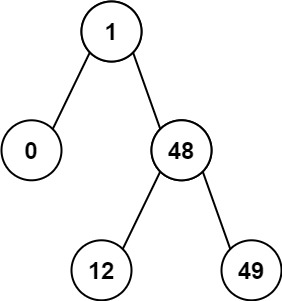### LeetCode• ㊗️
• 大家
• offer
• 多多！

## Problem

Given the root of a Binary Search Tree (BST), return the minimum absolute difference between the values of any two different nodes in the tree.

Example 1:Input: root = [4,2,6,1,3]
Output: 1


Example 2:Input: root = [1,0,48,null,null,12,49]
Output: 1


Constraints:

• The number of nodes in the tree is in the range [2, 10^4].
• 0 <= Node.val <= 10^5

Note: This question is the same as 783

## Code

class Solution {
Integer prev = null;
int diff = Integer.MAX_VALUE;

public int getMinimumDifference(TreeNode root) {
helper(root);
return diff;
}

private void helper(TreeNode root){
if(root == null) return;

helper(root.left);

if(prev == null){
prev = root.val;
} else {
diff = Math.min(diff, root.val - prev);
prev = root.val;
}

helper(root.right);
}
}